Lift

James M. Glownia December 14, 2007

(Submitted as coursework for Physics 210, Stanford University, Fall 2007)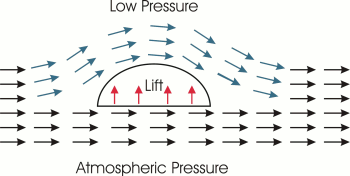Fig. 1: Airflow Over a Wing in Hump Theory.

The theory of aerodynamic lift and how airplanes fly is a topic often discussed in encyclopedias as well as in K-12 science textbooks . Unfortunately, many of these discussions present a physical model that is fundamentally incorrect, lacking either a common sense approach or a solid foundation in physical principles . Here we address this issue by first presenting a theory of lift commonly given to many students in grade school. To contrast with a more complete and accurate analysis, we follow this with a physically motivated discussion of lift and aerodynamics.

The "Hump"Theory

Many discussions of airplane lift utilize a vector field figure similar to the one in Fig. 1 first arguing that undisturbed air is split by the leading edge of the wing, with the air that is moving above the wing having a larger distance to travel than the air traveling along the bottom of the wing. The air itself is assumed to have equal transit times both above and below the wing and consequently air above the wing experiences an increase in velocity to keep pace with the air that is below the wing. The streamlines then merge once again at the trailing edge of the wing to reassume laminar flow. Bernoulli's Principle, given here as: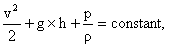defined with velocity (v), gravity(g), height (h), pressure (p), and density (rho), is applied where an increase in velocity (with a relatively negligible change in height) must correspondingly lead to a decrease in its pressure. Since the air flowing over the wing has higher velocity than the air below the wing, it must therefore have a relative lower pressure. As shown in Fig. 1, the resultant pressure difference between air above and below the wing results in a net upward force on the wing. Lift in this analysis can thus be seen due to simple suction similar to an air suction cup sticking to a window. To test this hypothesis, we present below a simple calculation using a commonly flown plane, namely a Boeing model 737-700.

Simple Hump Theory Based Calculation Estimating Lift

An empty Boeing 737-700 weighs roughly 40,000 kg, has a wingspan of 34.3 m, and a wing area of 125 m^2. In this example, the plane is at sea level with a takeoff velocity of 100 m/s. Neglecting the change in height in Bernoulli's Principle, the air velocity difference above and below the wing required to balance the plane's weight (here simply assumed distributed evenly over the wing area) is roughly 72 m/s. This analysis shows that the air above the wing needs to move roughly 50% farther than the air below the wing, essentially necessitating a 3-4 meter tall hump in the wing profile (roughly one story!). This clearly is not the case for actual airplanes, especially considering that this calculation has been done for an empty 737-700, whereas a loaded one can weigh up to 70,000 kg, which would make the hump even more absurdly severe. Clearly, there are flaws in this commonly applied physical interpretation of lift.

A Some Hump Theory Fundamental Issues

1. In the above analysis we see that the laminar stream lines split apart and then recombine to maintain laminar flow. Hence there is no net change in the vertical momentum of the air. This is in direct violation of Newton's Third Law of mechanics since for every action there must be an equal or opposite reaction. The reaction force of the air pushing up on the airplane wing should cause the air to have downward momentum, which is absent in this analysis.
2. There is no physical requirement that there be equal transit time for air above and below the wing, or that the application of Bernoulli's Principle is warranted since it requires that the fluid be incompressible (or streamlines of constant density) which is generally only the case for air at low velocities. In fact, studies have shown that the transit time for air above the wing is generally considerably shorter than below the wing.
3. Some stunt airplanes with symmetrical airfoils can fly very well upside down which would be impossible according to this theory. Many other ordinary planes can also fly upside down with simple adjustment of the wing attack angle.
4. Planes such as the Wright Brothers and paper gliders fly with only a single thin airfoil of cloth or paper that is nearly symmetrically shaped on the top and bottom. According to Hump theory, these planes could never fly due to nearly identical path lengths above and below the wings.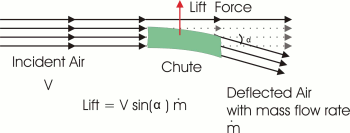Fig. 2: The Wing as a Chute.

Third Law Theory of Lift

The principles behind lift generated by wings are much more in common with a helicopter than say a suction cup. Lift works in any flying machine by having air blow downwards to create a subsequent reaction force to work against the force of gravity . In other words, an airfoil can be viewed as a chute that redirects air from a horizontal plane to a vertical plane as shown in Fig. 2. The act of bending moving air downwards requires that a net downward force be applied to the air by the chute. Consequently, according to Newton's Third Law, the chute itself must correspondingly experience a reaction force in the upward direction, and this is a realistic source of lift. It is natural to ask regarding the quantity of air that can be deflected by the wings, and why this air would necessarily even be deflected in the first place. These questions will be addressed in the following section.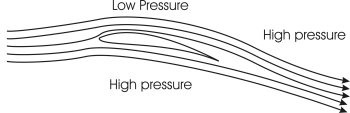Fig. 3: A More Realistic Streamline Diagram.

General Aerodynamics

Air is a viscous fluid and the dynamics of viscous fluid flow around objects is a key element in the development of lift. Molecules comprising a viscous fluid tend to stick to the surface of an encountered object. These molecules act to pull on neighboring fluid molecules which tends to retard them thus establishing a velocity gradient parallel to the object's surface normal as shown in the diagram. Such a velocity distribution that tends to pull the fluid around the contours of the object is a phenomenon known as the Coanda Effect. Hence if a curved airfoil is pointed downwards as shown in Fig. 3, air following the upper and lower surfaces of the wing would have a significant fraction of its velocity directed downwards as it leaves the trailing edge of the wing.

The downward curvature of the air by the Coanda Effect causes the air molecules to experience centripetal acceleration . The magnitude of the centripetal acceleration is governed by the Euler-n equation: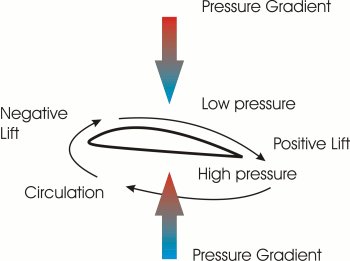Fig. 4: Pressure Gradients and Circulation Around the Wing.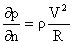where V is the velocity, R is the radius of curvature, dp is the differential pressure, and dn is the differential height of a small control volume. Clearly for a positive radius of curvature, such as on the upper surface of the wing, a gradient of low pressure is established that opposes atmospheric pressure. The result is decreased overall pressure near the top surface of the airfoil. Thus, part of the Hump theory presented earlier is indeed accurate, although this is not the precise origin of lift. Rather, this serves to explain why air is accelerated over the top surface of airfoils, since air moves from a high pressure area far ahead of the plane to a lower pressure regime over the wing, and then back to a high pressure area following the wing’s trailing edge . This fast moving air gives the enormous volumetric flow of air required to counter an airplane's relatively large mass. Further, air rapidly moving from higher pressure to lower pressure also encounters the viscous effects discussed earlier, causing circulation around the wing. This is another important aspect of lift.

In the case of the airfoil show in Fig. 4, a lower pressure is seen to be formed above the airfoil while a higher pressure is formed below the tailing edge of the airfoil. Higher pressure air is shown to move along the bottom of the airfoil, then up along the leading edge, and then back to the low pressure region along the top of the airfoil. This circulation actually creates negative lift, since air is being accelerated upwards around the wing's leading edge, and such effects must be considered and compensated for in an airplane's design. Forward recirculation is also the origin of the ground effect on lift. The ground limits the amount of air that can recirculate under a wing, causing the negative lift effect to be lessened, creating the illusion of increased positive lift.

Recirculation also occurs laterally on airfoils. Laterally recirculating air over the wing tip results from a relatively higher pressure below the wing and a lower pressure above the wing. Unfortunately, by disturbing the air boundary layer on the upper surface of the wing, these trailing vortices also lead to viscous drag and actually cause a net decrease in the airfoil's effective surface area. A vertical winglet can be installed on a wing's tip to help block such vortex formation which increases the overall lift area of a wing serving to make planes more energy efficient . Such winglets are in use and can be observed on many planes including some Boeing 737.

© 2007 James M. Glownia. The author grants permission to copy, distribute and display this work in unaltered form, with attribution to the author, for noncommercial purposes only. All other rights, including commercial rights, are reserved to the author.

References

 "lift." Encyclopœdia Britannica. 2007. Britannica Concise Encyclopedia. 12 Nov. 2007 .

 "What is Lift?" NASA Glenn Research Center. 2007. K-12 Aerodynamics. 3 Dec. 2007

 R. Fox and A. McDonald, Introduction to Fluid Mechanics (4th ed.) (Wiley, 1992).

 R. Turton, Principles of Turbomachinery (2nd ed.) (Chapman and Hall, 1995).

 J. Bertin and M. Smith. Aerodynamics for Engineers (2nd ed) (Prentice Hall, 1989).